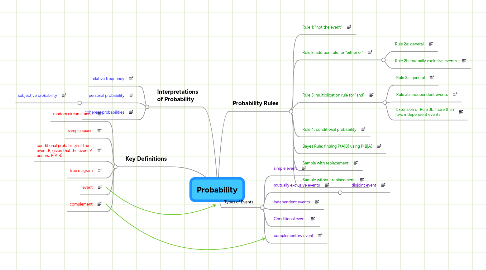# Probability

Get Started. It's FreeProbability## 1. Interpretations of Probability

### 1.2. personal probability

1.2.1. subjective probability

## 3. Probability Rules

### 3.2. Rule 2: addition rule for "either or"

3.2.1. Rule 2a: general

3.2.2. Rule 2b: mutually exclusive events

### 3.3. Rule 3: multiplication rule for "and"

3.3.1. Rule 3a: general

3.3.2. Rule 3b: independent events

3.3.3. Extension of Rule 3b: more than two independent events

## 4. Types of Events

### 4.2. mutually exclusive events

4.2.1. disjoint event